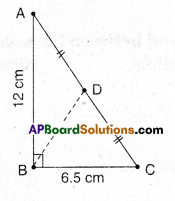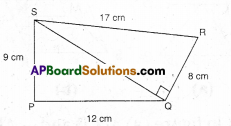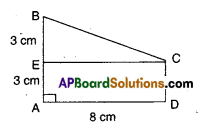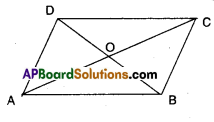# AP Board 9th Class Maths Solutions Chapter 11 Areas Ex 11.1

AP State Syllabus AP Board 9th Class Maths Solutions Chapter 11 Areas Ex 11.1 Textbook Questions and Answers.

## AP State Syllabus 9th Class Maths Solutions 11th Lesson Areas Exercise 11.1Question 1.
In ΔABC, ∠ABC = 90°; AD = DC; AB =12 cm, BC = 6.5 cm. Find the area of ΔADBSolution:
ΔADB = $$\frac { 1 }{ 2 }$$ ΔABC [ ∵ AD is a median of ΔABC]
$$\frac { 1 }{ 2 }$$ = [ $$\frac { 1 }{ 2 }$$ AB x BC]
= $$\frac { 1 }{ 4 }$$ x 12 x 6.5
= 19.5 cm2Question 2.
Find the area of a quadrilateral PQRS in which ∠QPS = ∠SQR = 90°, PQ = 12 cm, PS = 9 cm, QR = 8 cm and SR =17 cm.
[Hint: PQRS has two parts]Solution:
Area of ΔQPS = $$\frac { 1 }{ 2 }$$ x base x height
= $$\frac { 1 }{ 2 }$$ x 9 x 12
= 54cm2
In ΔQPS
QS2 = PQ2 + PS2
QS = \begin{aligned} \sqrt{12^{2}+9^{2}} &=\sqrt{144+81} \\ &=\sqrt{225}=15 \end{aligned}
Area of ΔQSR =$$\frac { 1 }{ 2 }$$ x base x height
= $$\frac { 1 }{ 2 }$$ x 15 x 8 = 60 cm2
∴ □PQRS = ΔQPS + ΔQSR
= 54 + 60= 114 cm2Question 3.
Find the area of trapezium ABCD as given in the figure in which ADCE is a rectangle.
[Hint: ABCD has two parts]Solution:
Area of trapezium 1
= $$\frac { 1 }{ 2 }$$ (sum of parallel sides) x (distance between the parallel sides)
= $$\frac { 1 }{ 2 }$$ (a + b) h
From the figure, a = 3 + 3 = 6 cm
b = 3 cm
(∵ Opp. sides of rectangle)
h = 8 cm
∴ A = $$\frac { 1 }{ 2 }$$(6 + 3)x8 = 36cm2Question 4.
ABCD is a parallelogram. The diago-nals AC and BD intersect each other at O. Prove that ar (ΔAOD) = ar (ΔBOQ. [Hint: Congruent figures have equal area]Solution:
Given that □ABCD is a parallelogram.
Diagonals AC and BD meet at ‘O’.
In ΔAOD and ΔBOC
AD = BC [ ∵ Opp. sides of a ||gm]
AO = OC [ ∵ diagonals bisect each
OD = OB other]
ΔAOD = ΔBOC [S.S.S. congruence]
∴ ΔAOD = ΔBOC (i.e., have equal area)